Mobile QR Code1. (System Integrated Circuit Design Lab, Inha University, 100, Inha-ro, Michuhol-gu, Incheon, Incheon 22212, Korea )

Decision feedback equalizer (DFE), unit interval (UI), time constraint, sign-sign least mean square (SS-LMS), four-level pulse amplitude modulation (PAM-4)

## I. INTRODUCTION

As 5G mobile communication technology becomes universalization and deep learning technology is applied to autonomous driving, visual recognition, demand for high data rate data transmission and receiving is increasing. As the data rate increases, the attenuation by the channel becomes higher and the resulting inter symbol interference (ISI) limits NRZ signaling). Thus, PAM-4 signaling capable of transmitting at twice the data rate is more attractive due to higher bandwidth efficiency than NRZ signaling (2,4).

In the data received through the transmission line, a reception error occurs due to a difference in attenuation between the low frequency and high frequency components. There are several equalization techniques to compensate for signal integrity. Continuous time linear equalization (CTLE) features a low power consuming and simple implementation and has the advantage of elimination both pre-cursor and post-cursor ISI. However, there is a limit to the frequency bandwidth due to a parasitic pole, and signal and noise increase by the same amount. Therefore, for improving the signal-to-noise ratio (SNR) characteristics, only ISI is selectively removed using a decision feedback equalizer (DFE) (1,2). DFE requires data sampling as well as the filter coefficient operation before the next sample, timing constraint is very tight on high-speed data rate. If the time constraint is not satisfied, the first tap cannot be used, and the ISI cannot be removed efficiently. There are two types of DFE, which are a direct DFE and a speculative DFE (6). The direct DFE removes ISI that appears in the next sample based on the preceding sample. Direct DFE structure uses the least number of the samplers but has the most stringent time constraint to meet. Various direct DFE have been proposed to reduce feedback delay, the same timing constraint still is applied (1,2,7). Speculative DFE selects the data with the highest reliability among the data judged by all cases (9). Since there is no feedback path, this structure is adopted to satisfy the given time constraint, but it uses too many samplers and multiplexers, especially in PAM4 signaling.

Fig. 1. (a) Direct, (b) Speculative DFE structure(full data rate cases for clarity).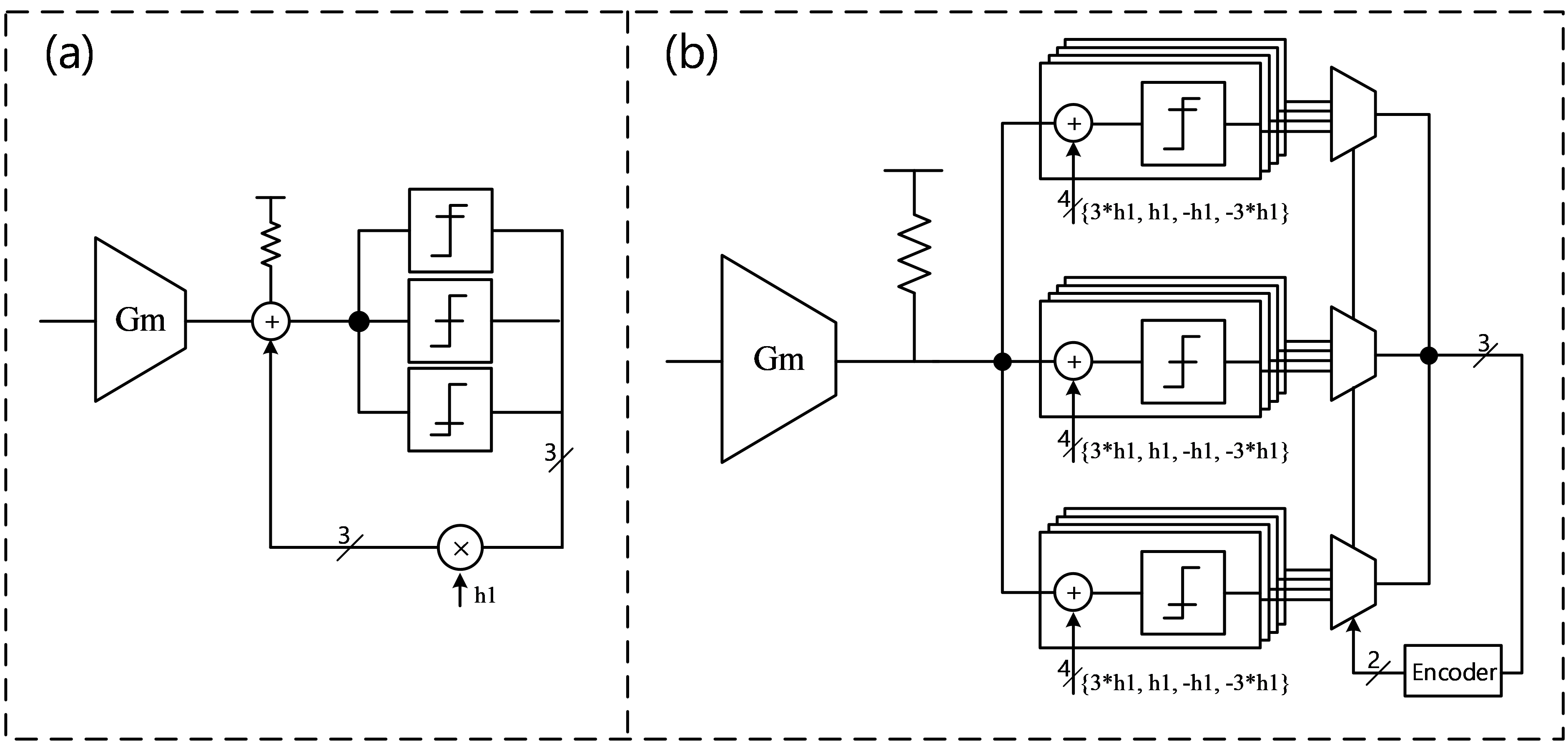This paper presents a novel approach to extend the time constraint of DFE to 1.5UI, which may replace the hardware consuming speculative DFE approach for PAM-4 signaling. Analysis, circuit structure, and simulation are described.

## II. PROPOSED DFE WITH EXTENDED TIME CONSTRAINT

### 1. Direct DFE and Speculative DFE

Fig. 1(a) shows a one tap direct DFE and Fig. 1(b) shows a one tap speculative DFE on a PAM-4 full data rate case for clarity. In the direct DFE structure, the time constraint of the critical path is given as

##### (1)
$\mathrm{T}_{\mathrm{clk}-\mathrm{q}}+\mathrm{T}_{\text {prop-vtoi }}+\mathrm{T}_{\text {sum-settle }}<1 \mathrm{UI}$

where $\mathrm{T}_{\mathrm{clk}-\mathrm{q}}$ is the clock-to-q delay of sampler, + $\mathrm{T}_{ ext {prop-vtoi }}$ is the propagation delay from the change in digitized $h1$ value to the change the current of the summer, and $\mathrm{T}_{ ext {sum-settle }}$ is settling time of summer output voltage in response to the summer current change. There is a speculative structure as a way to relax the time constraint in the direct DFE. The speculative structure does not require the settling time because ISI is not removed from the output node of the summer. However, the output nodes of summer need to drive 4x more samplers compared to the direct DFE. In other words, when multiplexers and encoders are included, 4 times more hardware is required than the direct DFE. Moreover, the loop created by the multiplexers to select a reliable value diminishes the advantage of speculative structure (2). In the speculative DFE structure, the time constraint of the critical path is given as

##### (2)
$\mathrm{T}_{\mathrm{clk}-\mathrm{q}}+\mathrm{T}_{\text {prop-mux }}<1 \mathrm{UI}$

where $\mathrm{T}_{\text {prop-mux }}$ is mux propagation delay. Since there are no feedback equalization actions through the summer, $\mathrm{T}_{ ext {prop-vtoi }}$ and $\mathrm{T}_{ ext {sum-settle }}$ are removed and only $\mathrm{T}_{\text {prop-mux }}$ is added. Therefore, it is easier for the speculative DFE to satisfy time constraint than direct structure.

### 2. Proposed DFE with Extended Time Constraint

A non-speculative adaptive DFE structure with a 1.5UI timing constraint is proposed. The proposed approach minimizes additional hardware while overcoming the drawbacks in direct DFE and speculative DFE. Fig. 2 shows a block diagram of proposed DFE for quarter-rate PAM-4 signaling. Compared to the conventional DFE structures, two samplers (DFE sampler and DATA sampler) were used. In preceding stage, a CTLE is employed to boost the high frequency components of the input signal. The output of CTLE needs to be tracked and held and a bootstrap structure of the track and hold (T&H) circuit is adopted in this work (1,8). T&H tracks output of CTLE for 2 UI on the falling edge of the clock and holds it for 2 UI on the rising edge of the clock. The output of T&H is equalized once again by DFE and then sampled by two types of samplers in different phases of clock. In direct DFE structure, only output of the data sampler is used as a tap coefficient, and it is encoded and used as recovered data. In proposed approach the role of DFE sampler and DATA sampler is different as described below. The output of the DFE sampler is used as a coefficient of tap and the output of the DATA sampler is encoded and used as data. A detailed timing diagram of the sampler is shown in Fig. 3. One T&H circuit tracks the input signal for 2UI from the falling edge of CLK270 and holds it for 2UI from the rising edge of CLK270. The output of this track and hold is shown as EYE270 in Fig. 3. EYE270 is sampled by the DFE sampler on the rising edge of CLK0 and by the DATA sampler on the rising edge of CLK45, respectively. The output of the DFE sampler by sampling EYE270 at CLK0 is used as the tap coefficient for equalizing EYE0. Equalized EYE0 is sampled by the DATA sampler at CLK135 and is used as recovered data. Since EYE0 is equalized by the output of the DFE sampler sampled at CLK0, it has 1.5UI timing margin from CLK0 to CLK135 for equalization. Therefore, 1UI time constraint of direct DFE can be extended to 1.5UI by separating the roles that one sampler was performing into DFE sampler and DATA sampler with different sampling timing. Eq. (3) represents the timing constraint of critical path from DFE sampler to DATA sampler, and Eq. (4) represents the timing constraint of critical path between two DFE samplers.

Fig. 3. Timing diagram about track and hold operation and sampling.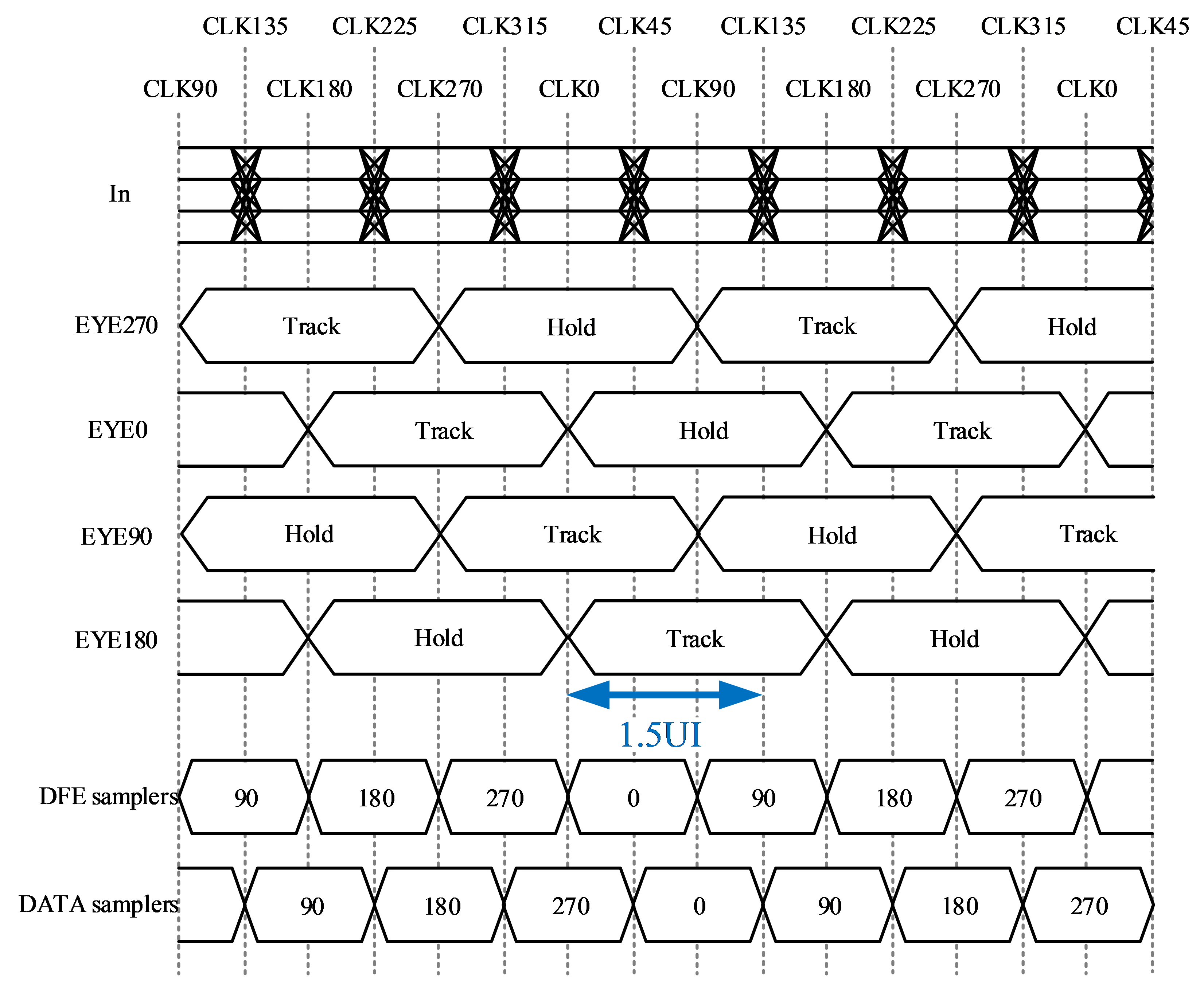##### (3)
$\mathrm{T}_{\text {clk-q }}+\mathrm{T}_{\text {prop-vtoi }}+\mathrm{T}_{\text {sum-settle }}<1.5 \mathrm{UI}$

##### (4)
$\mathrm{T}_{\mathrm{clk}-\mathrm{q}}+\mathrm{T}_{\text {prop-vtoi }}<1 \mathrm{UI}$

In order for the output voltage of summer is properly converged, the coefficient of tap must be completed within 0.5UI (3). Considering the case where equalization by tap has enough settling time, the time margin except the settling time is 0.5UI for the direct DFE and 1UI for the proposed DFE. Thus, the proposed DFE structure can take care of 2 times higher speed of data with enough settling time.

Table 1 and 2 show the values obtained by simulation of feedback delays for several data rates using a 65nm CMOS process when using strong-arm type latches and current mode logic (CML) type latches, respectively. Fig. 4 and 5 show the schematic of the strong-arm latch and CML-latch used to obtain the feedback delay (6,11). Table 1 shows that delay margin of the proposed DFE structure for 10 Gb/s input data is the same as that of 5 Gb/s in direct DFE structure. When using the strong-arm latch case, the direct DFE has an enough settling time up to 5 Gb/s data rate, while the proposed DFE structure can have an enough settling time up to 10 Gb/s data rate. In the case of the CML latch as shown in Table 2, input data rate with an enough settling time goes up from 15 Gb/s to 30 Gb/s. In the case of 7.5 Gb/s input data in Table 1, the 1UI time constraint has a negative delay margin of -0.16UI. That is, when the first tap with a direct DFE structure is implemented, it has a tap coefficient by the preceding data but always has insufficient settling time. Signals equalized by insufficient settling time have a relatively high probability of incorrect sampling output. This not only causes a bit error, but also causes an erroneous tap coefficient. In the case of the proposed 1.5UI structure, the delay margin is 0.34UI, which is sufficient for the settling time. That is, the signal equalized by the output of the DFE sampler is sampled by the DATA sampler with sufficient settling time. The DFE sampler has a time constraint of 1 UI, so it has insufficient settling time. Even if an error occurs in the output of the DFE sampler due to insufficient settling time, since the DATA sampler has sufficient settling time, a bit error does not occur. However, a problem occurs in the tap coefficient for the next sample. The output of the DATA sampler is more reliable than that of the DFE sampler because it has sufficient settling time. So, if an error occurs at the output of the DFE sampler, it is desirable to adjust the tap coefficient through the output of the DATA sampler. If the DFE sampler and the DATA sampler have the same value, the tap coefficient will have the correct value in advance by 0.5UI. And, if the DFE sampler and the DATA sampler have different outputs, i.e., if the output of the DFE sampler has an error, the tap coefficient is modified by the DATA sampler, so tap coefficient always has the correct value. That is, at 7.5 Gb/s signal, the direct DFE structure generate a bit error and tap coefficient error when the output of sampler is wrong. However, the proposed DFE structure, bit error does not occur because the DATA sampler has enough settling time. Also, because the tap coefficient is modified by the output of the DATA sampler when an error occurs in the output of DFE sampler, tap coefficient has always correct value. Likewise, in the case of 22.5 Gb/s in CML latch case as Table 2, the direct DFE has an insufficient settling time with a delay margin of -0.18UI, while the proposed DFE can implement a first tap with a sufficient settling time with a delay margin of 0.32UI. In Table 3, the number of samplers of the two conventional structures and the proposed structure are compared (2). Too many samplers increase the load capacitance of summer, which limits of the maximum equalization frequency range and excessively increasing power consumption. In PAM-4 signaling, the speculative structure requires 4 times more samplers of all types compare to the direct structure, while the proposed structure add only the DFE sampler to obtain extended time constraint. Table 4 shows the power consumption in each of the simulated cases above. As can be seen in Table 3, the proposed DFE can minimize the number of additional samplers, thus minimizing the additional power consumption compared to the speculative DFE.

Table 3. The number of samplers required according to the DFE structure (full-rate case)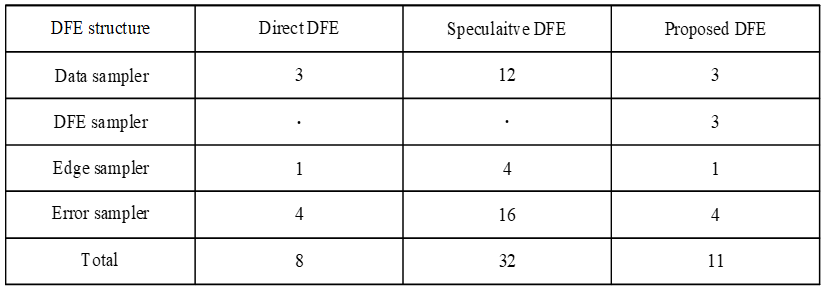### 3. DFE FIR Tap and Tap Weight Adaptation Algorithm

In general, DFE taps consist of NMOS taps (2,4). In the NMOS only tap, the stronger the current weight, the lower the common voltage of the signal. This causes several problems. First, too low common voltage reduces the gain and adversely affects the linearity of the summer, which have a critical effect on PAM4 signal with three eyes at the same time. Second, the sampler should be designed in consideration of the region of lower common voltage. Too low common voltage can change $\mathrm{T}_{\mathrm{clk}-\mathrm{q}}$, which causes a change in feedback delay. Finally, it causes a change in the threshold voltage of the PAM4 signal. The data level and threshold voltage determined based on the signal equalized by output of CTLE have different values by NMOS tap. This would require the re-establishment of data levels and threshold voltages. These issues would require a wide range of operating regions for summer and DAC, which would result in a mismatch. Thus, by using low voltage differential signaling (LVDS) tap, it is possible to keep the common voltage of the signal constant regardless of the weight through directional equalization (5).

##### (5)
$\mathrm{C}_{\mathrm{k}+1}=\mathrm{C}_{\mathrm{k}}+\Delta \operatorname{sign}\left[\varepsilon_{\mathrm{k}}\right] \operatorname{sign}\left[\mathrm{V}_{\mathrm{k}-1}\right]$

The circuit design of LVDS tap is given in Fig. 2. The current weight of the tap was controlled through the sign-sign least mean square (SS-LMS) algorithm. Eq. (5), C is the weight of tap, Δ is the step size of weight, $\operatorname{sign}\left[\varepsilon_{\mathrm{k}}\right]$ is the output of the error sampler, $\operatorname{sign}\left[\mathrm{V}_{\mathrm{k}-1}\right]$ is the output of the DATA sampler. The output of the DATA sampler specifies the $\operatorname{sign}\left[\varepsilon_{\mathrm{k}}\right]$ among 4 error samplers. The on and off of 3 taps is operated by the outputs of 3 DFE samplers and has the same coefficient.

Fig. 7. Eye diagram after CTLE when using channel 1 with 7.5 Gb/s input signal.Fig. 8. Eye diagram after CTLE when using channel 2 with 22.5 Gb/s input signal.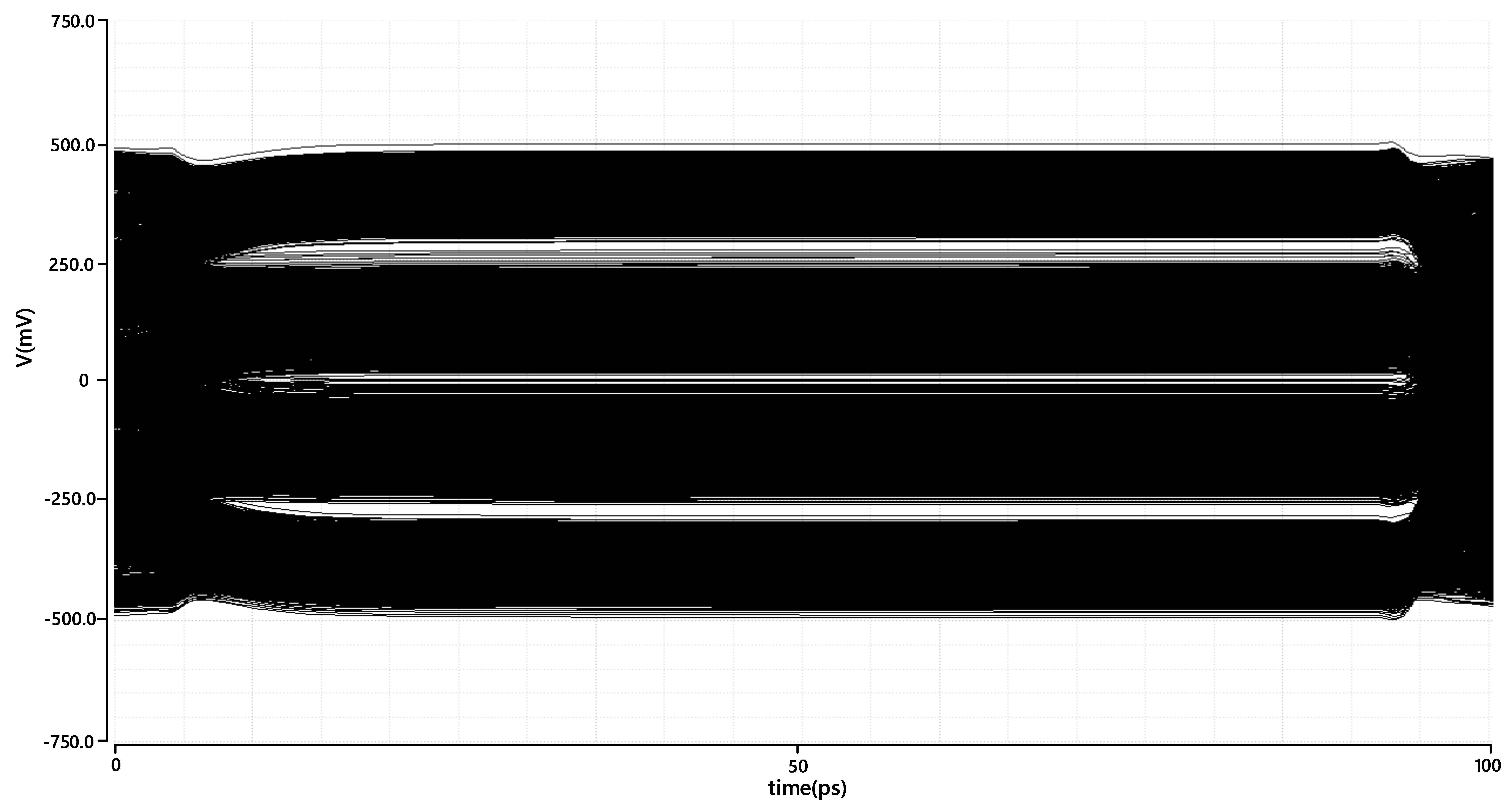## III. EXPERIMENTAL RESULTS

The proposed circuit was designed using 65 nm CMOS process and verified through simulation. PAM-4 PRBS pattern was used for input data, and Fig. 6 shows channel 1 (Ch1) with attenuation of 11.9 dB at 3.75 GHz and channel 2 (Ch2) with attenuation of 13.8 dB at 11.25 GHz. Fig. 7 and 8 shows the eye diagrams before equalization by DFE. Fig. 9 and 10 show eye diagrams with sampling point indication using Strong-arm latch at 7.5 Gbps with the direct DFE and the proposed DFE. Fig. 11 and 12 show eye diagrams with sampling point indication using CML latch at 22.5 Gbps with the direct DFE and the proposed DFE. Fig. 9 and 11 show that sampling is performed at a less stabilized point. So, the eye height is not enough. On the other hand, Fig. 10 and 12 show that DATA sampling is performed with enough settling time, so the eye height for DATA sampler is larger than using direct DFE. Fig. 13 shows that the current tap weight was stabilized using the LVDS tap. Since LVDS tap must be activated without changing the common mode of the signal, the current through the NMOS tap and the PMOS tap need to be the same. Therefore, the weights of the NMOS current source and the PMOS current source operate symmetrically, taking into account the threshold voltage of MOSFET.

Fig. 9. Eye diagram when using strong-arm latch in Direct DFE.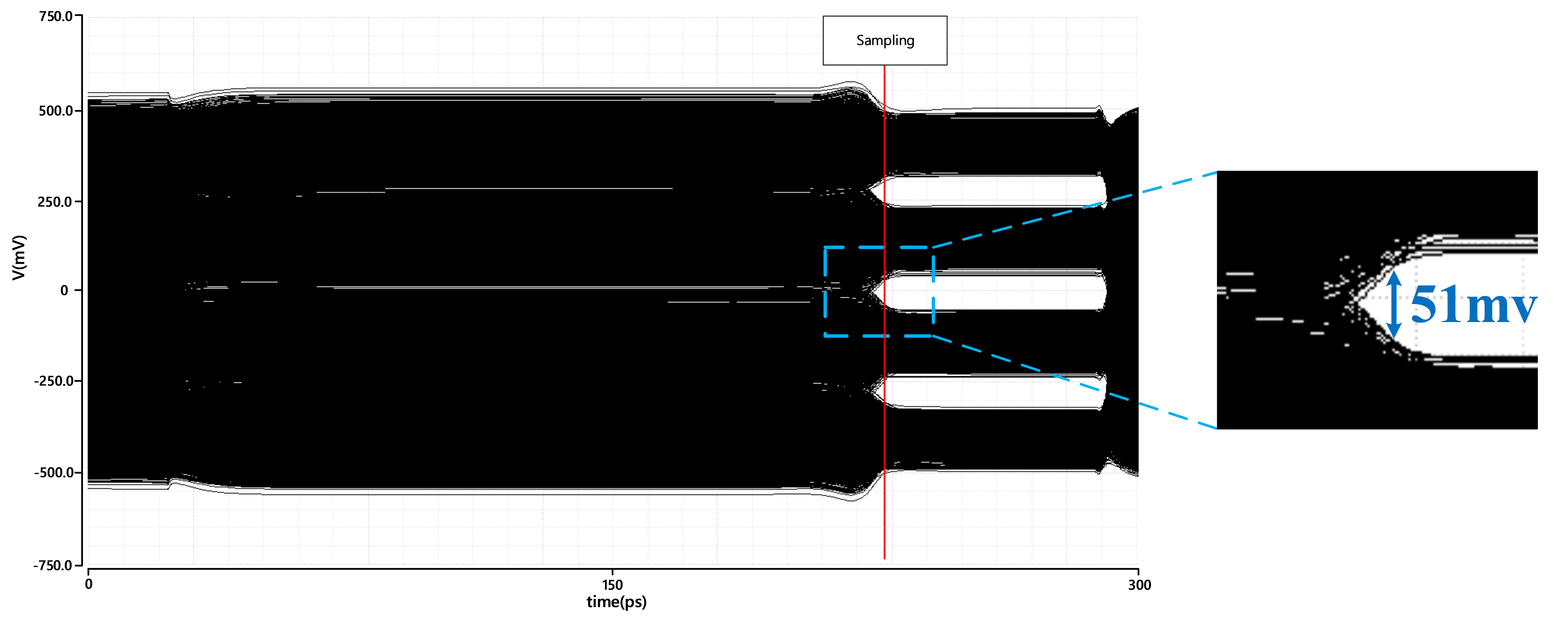Fig. 10. Eye diagram when using strong-arm latch in proposed DFE.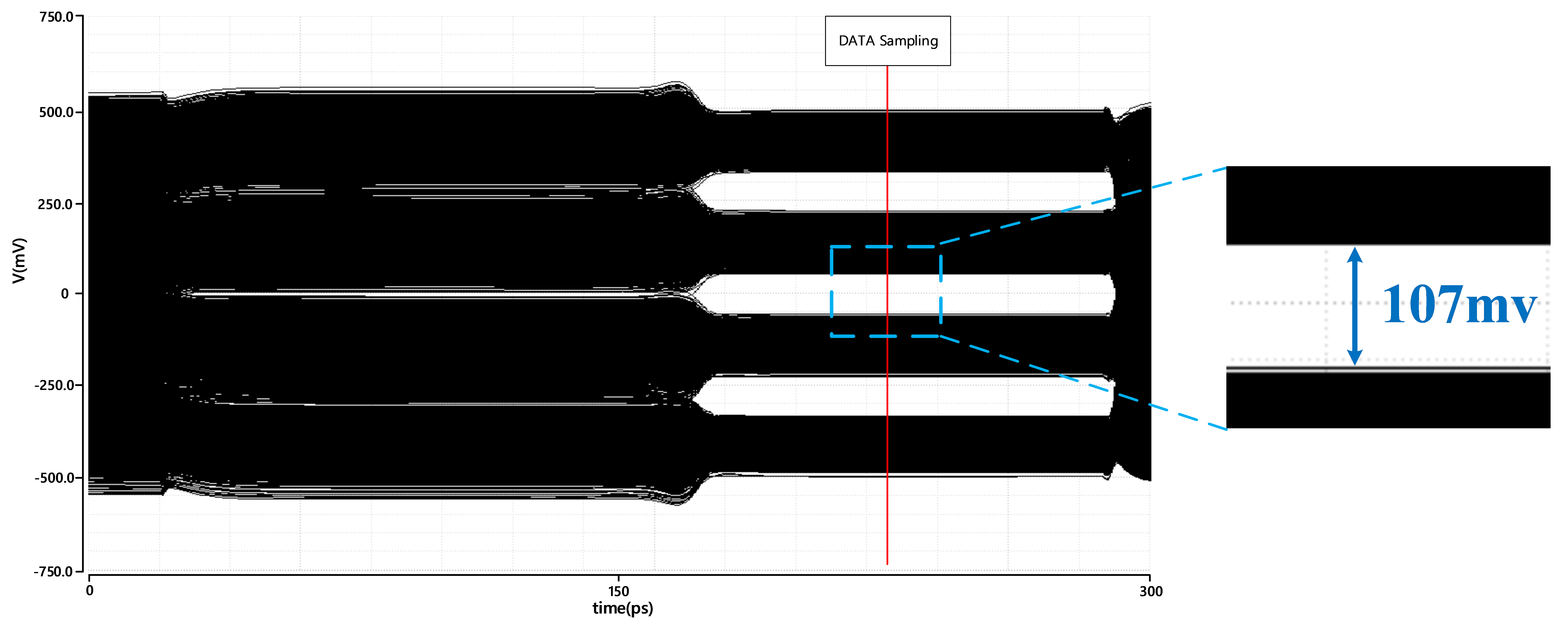Fig. 12. Eye diagram when using CML latch in proposed DFE.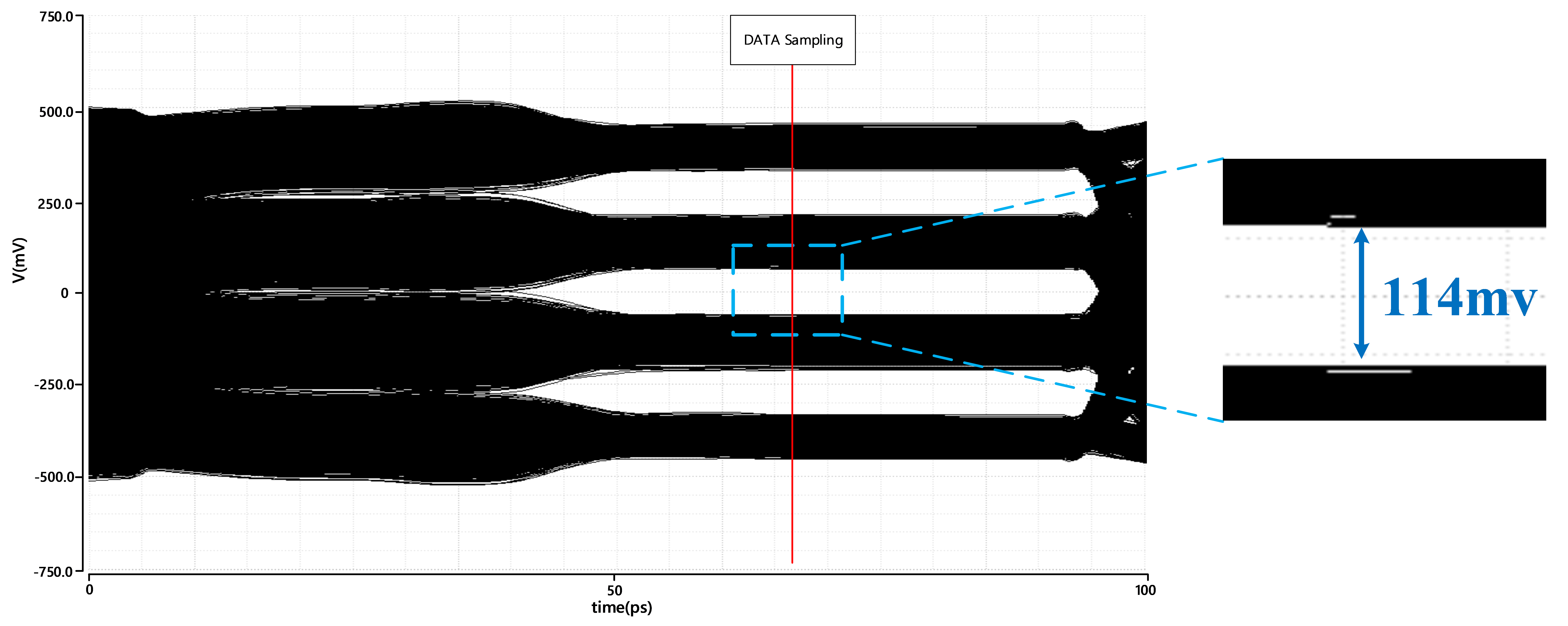## V. CONCLUSIONS

In this paper, a non-speculative DFE with a time constraint of 1.5 UI was proposed. The proposed DFE requires only the DFE sampler additionally and has a time constraint similar to that of the PAM-4 speculative DFE, which requires 4 times more hardware in the summer output node than the direct DFE. The improved time constraint through the proposed structure shows that the DFE implemented with the first tap can operate stably with sufficient settling time at 7.5 Gb/s and 22.5 Gb/s, respectively.

### ACKNOWLEDGMENTS

This research was supported by National R&D Program through the National Research Foundation of Korea(NRF) funded by Ministry of Science and ICT(No. 2020M3H2A1076786) and the MOTIE (Ministry of Trade, Industry & Energy (10080285) and KSRC (Korea Semiconductor Research Consortium) support program for the development of the future semiconductor device. Authors also thank the IDEC program and for its hardware and software assistance for the design and simulation.

### REFERENCES

1
Roshan-Zamir A., Elhadidy O., Yang H., Palermo S., Sept. 2017, A Reconfigurable 16/32 Gb/s Dual-Mode NRZ/PAM4 SerDes in 65-nm CMOS, Solid-State Circuits, IEEE Journal of, Vol. 52, No. 4, pp. 2430-24472
Im J., Dec 2017, A 40-to-56 Gb/s PAM-4 Receiver With Ten-Tap Direct Decision-Feedback Equalization in 16-nm FinFET, Solid-State Circuits, IEEE Journal of, Vol. 52, No. 12, pp. 3486-35023
Payne R., Dec 2005, A 6.25-Gb/s binary transceiver in 0.13-/spl mu/m CMOS for serial data transmission across high loss legacy backplane channels, Solid-State Circuits, IEEE Journal of, Vol. 40, No. 12, pp. 2646-26574
Roshan-Zamir A., Mar 2019, A 56-Gb/s PAM4 Receiver With Low-Overhead Techniques for Threshold and Edge-Based DFE FIR- and IIR-Tap Adaptation in 65-nm CMOS, Solid-State Circuits, IEEE Journal of, Vol. 54, No. 3, pp. 672-6845
Dolan M., Yuan F., MA. 2017, An adaptive edge decision feedback equalizer with 4PAM signaling, Circuit and Systems, 2017, MWSCAS 2017, 60th IEEE International Midwest Symposium on, pp. 535-5386
Fei YUAN, 2014, Design techniques for decision feedback equalization of multi-giga-bit-per-second serial data links: a state-of-the-art review, Devices & Systems, 2014, IET Circuits, Vol. 8, pp. 118-1307
Chen K., Chen W., Liu S., Nov 2017, A 0.31-pJ/bit 20-Gb/s DFE With 1 Discrete Tap and 2 IIR Filters Feedback in 40-nm-LP CMOS, Circuits and Systems II : IEEE Transactions on, Vol. 64, No. 11, pp. 1282-12868
Krupnik Y., IEEE Journal of, 112-Gb/s PAM4 ADC-Based SERDES Receiver with Resonant AFE for Long-Reach Channels, Solid-State Circuits, IEEE Journal of, Vol. 55, No. 4, pp. 1077-10859
Li Y., Yuan F., MA. 2017, Adaptive data-transition decision feedback equalizer for serial links, Circuit and Systems, 2017, MWSCAS 2017, 60th IEEE International Midwest Symposium on, pp. 1609-161210
Chen K. -C., Kuo W. W. -T., Emami A., Mar 2021, A 60-Gb/s PAM4 Wireline Receiver With 2-Tap Direct Decision Feedback Equalization Employing Track-and-Regenerate Slicers in 28-nm CMOS, Solid-State Circuits, IEEE Journal of, Vol. 56, No. 3, pp. 750-76211
Jung J. W., Razavi B., Feb 2015, A 25 Gb/s 5.8 mW CMOS Equalizer, Solid-State Circuits, IEEE Journal of, Vol. 50, No. 2, pp. 515-52612
LEE J., Chiang P., Peng P., Chen L., Weng C., Sep 2015, Design of 56 Gb/s NRZ and PAM4 SerDes Transceivers in CMOS Technologies, Solid-State Circuits, IEEE Journal of, Vol. 50, No. 9, pp. 2061-2073## Author

Do-Hyeon Kwon received the B.S. degree in Electronic Engineering from Inha University, Incheon, South Korea, in 2020.

He is currently pursuing the M.S degree in Electrical and Computer Engineering with Inha University.

His research interests include transmitter and receiver equalizer design for high-speed serial interface.

Hyung-Wook Lee received the B.S degree in electronic engineering from Inha University, Incheon, South Korea, in 2020.

He is currently pursuing the M.S degree in Electrical and Computer Engineering with Inha University.

His research interests include high-speed serial interface and reference-less clock and data recovery circuit.

Kyeong-Min Ko received the B.S degree in electronic engineering from Inha University, Incheon, South Korea, in 2020.

He is currently pursuing the M.S degree in Electrical and Computer Engineering with Inha University.

His research interests include transmitter design for PAM4 signaling.

Taek-Joon An received the B.S and M.S degrees in Electrical Engi-neering from Inha University, Incheon, South Korea, in 2007 and 2014, respectively, where he is currently pursuing the Ph.D. degree in Electrical and Computer Engi-neering.

Jin-Ku Kang received the Ph.D. in electrical and computer engineering from North Carolina State University, Raleigh, NC, USA, in 1996.

From 1983 to 1988, he was with Samsung Electronics, Inc., Korea, where he was involved in memory design.

In 1988, he was with Texas Instrument in Korea.

From 1996 to 1997, he was with Intel Corp., Portland, OR, USA as a senior design engineer, where he was involved in high-speed I/O and timing circuits for processors.

Since 1997, he has been with Inha University, Department of Electronics Engineering, in Incheon, Korea.

His research interests include high-speed/low-power mixed-mode circuit design and prototyping with FPGA for high-speed serial interfaces.